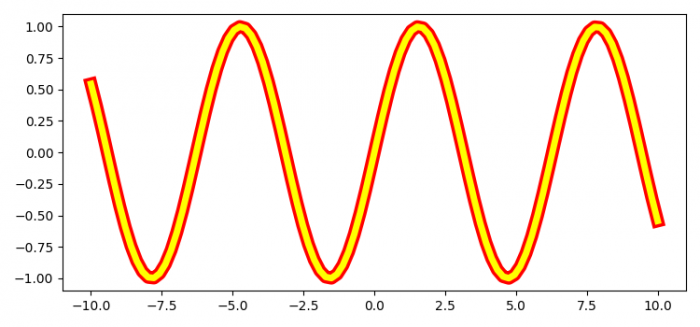# How to plot the outline of the outer edges on a Matplotlib line in Python?

MatplotlibPythonData Visualization

To plot the outline of the outer edges on a Matplotlib in Python, we can take the following steps −

• Set the figure size and adjust the padding between and around the subplots.
• Create x and y data points using numpy.
• Plot x and y data points with linewidth set to 10 and 5, to get the visible outline edges.
• To display the figure, use show() method.

## Example

import numpy as np
from matplotlib import pyplot as plt

plt.rcParams["figure.figsize"] = [7.50, 3.50]
plt.rcParams["figure.autolayout"] = True

x = np.linspace(-10, 10, 100)
y = np.sin(x)

plt.plot(x, y, lw=10, color='red')
plt.plot(x, y, lw=5, color='yellow')

plt.show()

## Output